CELIA3D  1.0 Fluid-structure interaction using cut-cells
Particule Class Reference

Particule class. More...

#include <solide.hpp>

## Public Member Functions

Particule ()
Default constructor. More...

Particule (const double x_min, const double y_min, const double z_min, const double x_max, const double y_max, const double z_max)

Particule (Point_3 c, const double x_min, const double y_min, const double z_min, const double x_max, const double y_max, const double z_max, std::vector< Face > &F)

~Particule ()
Destructor. More...

Particuleoperator= (const Particule &P)

void Affiche ()
Test display function. More...

double volume ()
Test function to check the conservation of the volume of the particle. More...

void CompVolumeIntegrals (double &T1, double &Tx, double &Ty, double &Tz, double &Txx, double &Tyy, double &Tzz, double &Txy, double &Tyz, double &Tzx)
Computation of volume integrals. More...

void Inertie ()
Computation of the particle inertia matrix. More...

void Volume_libre ()
Computation of the free volume. More...

void solve_position (double dt)
Time-integration of the particle position. More...

void solve_vitesse (double dt)
Time-integration of the particle velocity. More...

Vector_3 vitesse_parois (const Point_3 &X_f)
Velocity of the solid at the interface elements center at time t.
. More...

Vector_3 vitesse_parois_prev (const Point_3 &X_f)
Velocity at the interface elements center at time t-dt.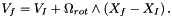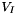is the particle velocity (Particule.u_half),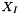is the particle center (Particule.x0 + Particule.Dxprev),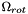is the particle rotation (Particule.omega_half). More...

## Data Fields

bool cube
= true if the particle is a cube, false otherwise More...

Bbox bbox
Bounding box of the particle. More...

std::vector< Facefaces
List of the particle's faces. More...

std::vector< Point_3vertices
List of the particle's vertices. More...

Triangles triangles
Triangulation of the particle faces at time t. More...

Triangles triangles_prev
Triangulation of the particle faces at time t-dt. More...

std::vector< Vector_3normales
Exterior normals to Particule.triangles. More...

std::vector< Vector_3normales_prev
Exterior normals to Particule.triangles_prev. More...

std::vector< bool > vide
=true if Particule.triangles is in contact with void More...

std::vector< bool > fluide
=true if Particule.triangles is in contact with fluid More...

std::vector< bool > fluide_prev
=true if Particule.triangles_prev is in contact with fluid More...

std::vector< std::vector< Point_3 > > Points_interface
Listof intersection points of Particule.triangles with the fluid grid at time t. More...

std::vector< std::vector< Point_3 > > Points_interface_prev
List of intersection points of Particule.triangles_prev with the fluid grid at time t-dt. More...

std::vector< std::vector< Triangle_3 > > Triangles_interface
Triangulation of Particule.triangles at time t. More...

std::vector< std::vector< std::vector< int > > > Position_Triangles_interface
Index of the cell where Triangles_interface is located at time t. More...

std::vector< std::vector< Triangle_3 > > Triangles_interface_prev
Triangulation of Particule.triangles_prev at time t-dt. More...

std::vector< std::vector< std::vector< int > > > Position_Triangles_interface_prev
Index of the cell where Triangles_interface is located at time t-dt. More...

int fixe
=1 if the particle is fixed, 0 otherwise More...

double m
Particle mass. More...

double V
Particle volume. More...

double Vl
Free Volume of the particle (for the computation of epsilon) More...

double epsilon
Volumetric deformation of the particle. More...

double I 
Inertia matrix of the particle. More...

double rotref 
Rotation matrix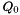such that the inertia matrix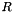in the reference frame can be written :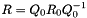, with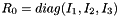. More...

Point_3 x0
Position of the particle center at t=0. More...

Vector_3 Dx
Displacement of the particle center at time t. More...

Vector_3 Dxprev
Displacement of the particle center at time t-dt. More...

Vector_3 Fi
Solid internal forces. More...

Vector_3 Ff
Fluid forces applied on the solid between times t and t+dt/2. More...

Vector_3 Ffprev
Fluid forces applied on the solide between times t-dt/2 and t. More...

Vector_3 Mi
Interior torques of the solid. More...

Vector_3 Mf
Fluid torques applied on the solid between times t and t+dt/2. More...

Vector_3 Mfprev
FLuid torques applied on the solid between times t-dt/2 and t. More...

Vector_3 u
Particle velocity at time t. More...

Vector_3 u_half
Particle velocity at time t-dt/2. More...

Vector_3 omega
Angular velocity at time t. More...

Vector_3 omega_half
Angular velocity at time t-dt/2. More...

Vector_3 e
Rotation vector at time t. More...

Vector_3 eprev
Rotation vector at time t-dt. More...

Aff_transformation_3 mvt_t
Affine transformation associated with the rigid body movement of the particle at time t. More...

Aff_transformation_3 mvt_tprev
Affine transformation associated with the rigid body movement of the particle at time t-dt. More...

Particule class.

## Constructor & Destructor Documentation

 Particule::Particule ( )

Default constructor.

 Particule::Particule ( const double x_min, const double y_min, const double z_min, const double x_max, const double y_max, const double z_max )

Parameters
 (x_min,y_min,z_min),(x_max,y_max,z_max) bounding box coordinates
 Particule::Particule ( Point_3 c, const double x_min, const double y_min, const double z_min, const double x_max, const double y_max, const double z_max, std::vector< Face > & F )

Parameters
 (x_min,y_min,z_min),(x_max,y_max,z_max) Bounding box coordinates c Point F Face of the Particule
 Particule::~Particule ( )

Destructor.

## Member Function Documentation

 void Particule::Affiche ( )

Test display function.

 void Particule::CompVolumeIntegrals ( double & T1, double & Tx, double & Ty, double & Tz, double & Txx, double & Tyy, double & Tzz, double & Txy, double & Tyz, double & Tzx )

Computation of volume integrals.

Uses the function described by Brian Mirtich (1996).

Returns
void
 void Particule::Inertie ( )

Computation of the particle inertia matrix.

Returns
void
 Particule & Particule::operator= ( const Particule & P )

Parameters
Returns
Particule
 void Particule::solve_position ( double dt )

Time-integration of the particle position.

Parameters
 dt Time-step
Warning
Uses Particule.Ff and Particule.Mf (fluid forces and torques moments fluides applied on the solid between times t and t+dt/2)
Particule.Ff and Particule.Mf are specific coupling parameters !
Returns
void
 void Particule::solve_vitesse ( double dt )

Time-integration of the particle velocity.

Parameters
 dt Time-step
Warning
Use of Particule.Ff and Particule.Mf (fluid forces and torques applied on the solid between times t and t+dt)
Particule.Ff and Particule.Mf are specific coupling parameters !
Returns
void
 Vector_3 Particule::vitesse_parois ( const Point_3 & X_f )

Velocity of the solid at the interface elements center at time t.
.is the particle velocity (Particule.u_half),is the particle center (Particule.x0 + Particule.Dx),is the particle rotation (Particule.omega_half).

Parameters
 X_f interface element center
Warning
Specific coupling procedure !
Returns
Vector_3
 Vector_3 Particule::vitesse_parois_prev ( const Point_3 & X_f )

Velocity at the interface elements center at time t-dt.is the particle velocity (Particule.u_half),is the particle center (Particule.x0 + Particule.Dxprev),is the particle rotation (Particule.omega_half).

Parameters
 X_f interface element center
Warning
Specific coupling procedure !
Returns
Vector_3
 double Particule::volume ( )

Test function to check the conservation of the volume of the particle.

Returns
double
 void Particule::Volume_libre ( )

Computation of the free volume.

Returns
void

## Field Documentation

 Bbox Particule::bbox

Bounding box of the particle.

 bool Particule::cube

= true if the particle is a cube, false otherwise

 Vector_3 Particule::Dx

Displacement of the particle center at time t.

 Vector_3 Particule::Dxprev

Displacement of the particle center at time t-dt.

 Vector_3 Particule::e

Rotation vector at time t.

 Vector_3 Particule::eprev

Rotation vector at time t-dt.

 double Particule::epsilon

Volumetric deformation of the particle.

 std::vector Particule::faces

List of the particle's faces.

 Vector_3 Particule::Ff

Fluid forces applied on the solid between times t and t+dt/2.

Warning
Specific coupling parameter !
 Vector_3 Particule::Ffprev

Fluid forces applied on the solide between times t-dt/2 and t.

Warning
Specific coupling parameter !
 Vector_3 Particule::Fi

Solid internal forces.

 int Particule::fixe

=1 if the particle is fixed, 0 otherwise

 std::vector Particule::fluide

=true if Particule.triangles is in contact with fluid

Warning
Specific coupling parameter !
 std::vector Particule::fluide_prev

=true if Particule.triangles_prev is in contact with fluid

Warning
Specific coupling parameter !
 double Particule::I

Inertia matrix of the particle.

 double Particule::m

Particle mass.

 Vector_3 Particule::Mf

Fluid torques applied on the solid between times t and t+dt/2.

Warning
Specific coupling parameter !
 Vector_3 Particule::Mfprev

FLuid torques applied on the solid between times t-dt/2 and t.

Warning
Specific coupling parameter !
 Vector_3 Particule::Mi

Interior torques of the solid.

 Aff_transformation_3 Particule::mvt_t

Affine transformation associated with the rigid body movement of the particle at time t.

 Aff_transformation_3 Particule::mvt_tprev

Affine transformation associated with the rigid body movement of the particle at time t-dt.

 std::vector Particule::normales

Exterior normals to Particule.triangles.

 std::vector Particule::normales_prev

Exterior normals to Particule.triangles_prev.

 Vector_3 Particule::omega

Angular velocity at time t.

 Vector_3 Particule::omega_half

Angular velocity at time t-dt/2.

 std::vector< std::vector > Particule::Points_interface

Listof intersection points of Particule.triangles with the fluid grid at time t.

Warning
Specific coupling parameter !
 std::vector< std::vector > Particule::Points_interface_prev

List of intersection points of Particule.triangles_prev with the fluid grid at time t-dt.

Warning
Specific coupling parameter !
 std::vector< std::vector< std::vector > > Particule::Position_Triangles_interface

Index of the cell where Triangles_interface is located at time t.

Warning
Specific coupling parameter !
 std::vector< std::vector > > Particule::Position_Triangles_interface_prev

Index of the cell where Triangles_interface is located at time t-dt.

Warning
Specific coupling parameter !
 double Particule::rotref

Rotation matrixsuch that the inertia matrixin the reference frame can be written :, with.

 Triangles Particule::triangles

Triangulation of the particle faces at time t.

 std::vector< std::vector > Particule::Triangles_interface

Triangulation of Particule.triangles at time t.

Warning
Specific coupling parameter!
 std::vector< std::vector > Particule::Triangles_interface_prev

Triangulation of Particule.triangles_prev at time t-dt.

Warning
Specific coupling parameter !
 Triangles Particule::triangles_prev

Triangulation of the particle faces at time t-dt.

 Vector_3 Particule::u

Particle velocity at time t.

 Vector_3 Particule::u_half

Particle velocity at time t-dt/2.

 double Particule::V

Particle volume.

 std::vector Particule::vertices

List of the particle's vertices.

 std::vector Particule::vide

=true if Particule.triangles is in contact with void

Warning
Specific coupling parameter !
 double Particule::Vl

Free Volume of the particle (for the computation of epsilon)

 Point_3 Particule::x0

Position of the particle center at t=0.

The documentation for this class was generated from the following files: